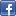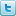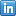# Adding User-Defined 'C' Routines to Real-Time DSP Code

Note: This section assumes you are familar with the concept of DSP Source Code Interfaces, and know the basic process of modifying Hypersignal DSP Source Code.

Following is general information about how to add user-defined 'C' routines to real-time DSP code running under Hypersignal®-Macro or Hypersignal-Acoustic software (or DirectDSP® software when Hypersignal is used as the "DSP engine"). Actual 'C' code examples are given; differences between DSP chip families are accounted for with #ifdef..#endif constructs.

### Overview

There are four (4) important ideas underlying the method used to add user-defined 'C' routines to real-time DSP code:

• hide details of the DSP chip and DSP board--especially the analog I/O, which tends to vary dramatically from board to board and can be extremely complex from a software point of view

• allow user-defined 'C' code to process analog input/output data on a buffer, or "frame" basis, and not deal with individual samples

• allow real-time recording of intermediate results to waveform file or memory for debugging purposes

• make the modification cycle, or "turn-around time", as fast as possible

To achieve these goals, a routine called "userproc.c" has been defined in standard Hypersignal DSP Source Code interfaces which represents a general entry-point for user-defined 'C' code. UserProc is called after each analog input/output buffer combination is processed by the analog I/O drivers and has been presented to the foreground processing layer in the DSP operating system. This occurs before analog input buffers are sent to host PC software, such as Hypersignal, or Windows programs using DirectDSP DLL software (e.g. application-specific C/C++, Visual Basic, or MATLAB programs). In this way, both analog input buffers (before they reach host PC applications) and output buffers (after they are sent by host PC applications) can be modified by real-time DSP code.

### UserProc: Real-Time 'C' Code Entry Point

Userproc.c represents a general entry-point for user-defined 'C' routines. It is called after each analog input/output buffer combination is processed by the analog I/O drivers and has been presented to the foreground processing layer in the DSP operating system. This type of operation is known as "frame-based real-time processing", where background interrupt service routines use automatic serial port DMA onchip circuitry to assemble analog input samples into a series of frames, or buffers, which are then processed to the foreground algorithm for processing on a "frame" basis. Note that the "current analog I/O drivers" are drivers that have been selected in the host PC software user-interface for the DSP/analog hardware that is currently installed in the system. In Hypersignal software this is done using the System Config menu selection; in DirectDSP software this is done using the DSP/Analog Hardware Configuration dialog box.

Parameters passed to userproc.c include a pointer to the just-acquired input buffer, a pointer to the next output buffer, length of each buffer in elements, and number of traces (channels) contained in each element. Note that each "element" could actually be more than one sample; for example a three-channel acquisition would cause buffers to contain interleaved data in the form ch0, ch1, ch2, ch0, ch1, ch2, etc.

Below is the default source code listing for the basic userproc.c routine, which is configured for simple loopback operation (loop input back to output) before user-defined modification:

Default UserProc Routine

```void UserProc(void* ptrIn, void* ptrOut, long nLen, short int nNumTrace) {

#ifdef defined(TMS320C3x) || defined(TMS320C4x) || defined(DSP5600x) || defined(ADSP2106x)
#define x ((long*)ptrIn)
#define y ((long*)ptrOut)
#endif

#ifdef defined(DSP32C) || defined(DSP3210) || defined(TMS320C2x) || defined(TMS320C5x)
#define x ((short int*)ptrIn)
#define y ((short int*)ptrOut)
#endif

short int  n;

for (n=0; n<nLen; n++) {

y[n] = x[n];  /* loop input back to output */

#ifdef defined(TMS320C3x) || defined(TMS320C4x)
y[n] <<= 16;
#endif
}
}
```

Here are some important notes about the above code structure:

1) Each input buffer ("x") contains 16-bit A/D values represented as right-justified, signed, N-bit integer values, where N is the natural integer word-length of the DSP device. The only exception to this are DSP32C and DSP3210 devices, in which case input buffer values are packed as 16-bit signed integers, even though these devices are 32-bit floating-point (they can make 8-bit, 16-bit, or 32-bit memory accesses).

2) Each output buffer ("y") contains 16-bit D/A values represented as right-justified, signed, N-bit integer values, where N is the natural integer word-length of the DSP device. Exceptions include DSP32C and DSP3210 devices, in which case output buffer values are packed as 16-bit signed integers, and TMS320C3x and TMS3204x devices, in which case output buffer values are left-justified 16-bit D/A values in a 32-bit integer. The TMS320xx exception is due to Hypersignal operation which historically has used "upper half" writes to obtain fast transfer of data for older 'C3x boards with 16-bit host PC interfaces. For several of these boards, a single 16-bit write was the fastest way to send D/A output data, but only the upper 16 bits could be accessed this way.

3) The nNumTrace parameter usually corresponds to the number of channels. However, it is more accurate to say "traces" instead of "channels" because the Channel List specified in Hypersignal or DirectDSP software may actually be an arbitrary ordering of channels, in which case, for example, channel 0 may not correspond to trace 0.

If the nNumTrace parameter is > 1, then data is stored as interleaved values, in repeating groups of nNumTrace values. For example,

`    tr0,tr1,tr2,tr0,tr1,tr2, ...`

for 3-trace acquisition or signal output.

5) Important: UserProc must complete its processing before the next input/output buffer pair is ready! Otherwise, real-time operation is lost.

### Application Examples

A few simple application examples are given below.

Signal Arithmetic Example

A very simple example of user-defined real-time 'C' code is basic signal arithmetic requiring no recursive input (i.e. no input from previous output samples). In this example, the DSP algorithm scales and adds a constant to input channel 0 data, and also loops the data back to the output. The difference equation for this example could look something like:

`    y[n] = 0.75*x[n] + 1000`

First, userproc.c is modified to look like:

```void UserProc(void* ptrIn, void* ptrOut, long nLen, short int nNumTrace) {

#ifdef defined(TMS320C3x) || defined(TMS320C4x) || defined(DSP5600x) || defined(ADSP2106x)
#define x ((long*)ptrIn)
#define y ((long*)ptrOut)
#endif

#ifdef defined(DSP32C) || defined(DSP3210) || defined(TMS320C2x) || defined(TMS320C5x)
#define x ((short int*)ptrIn)
#define y ((short int*)ptrOut)
#endif

short int  n;

for (n=0; n<nLen; n++) {

x[n] = 0.75*x[n] + 1000;
y[n] = x[n];

#ifdef defined(TMS320C3x) || defined(TMS320C4x)
y[n] <<= 16;
#endif
}
}
```

Second, the following steps are performed:

Running Sum Example

A slightly more complex example is to combine basic signal arithmetic with recursive input (i.e. input from previous output samples). In this example, the DSP algorithm performs a running sum on input channel 0 data, with no loopback to the output. The difference equation for this example could look something like:

`    y[n] = y[n-1] + (x[n] - x[n-M])/M`

where M is the length of the running sum, in samples. First, userproc.c is modified to look like:

```#define N 4096  /* define N as maximum buffer size */
#define M 32    /* define M as length of running sum */

short int ySum[N];    /* create storage of size of one buffer, assume it is initialized
to zero */

short int xPrev[M];   /* create storage of length of running sum, to hold samples at end
of each
previous buffer ("memory" between successive frames) */

void UserProc(void* ptrIn, void* ptrOut, long nLen, short int nNumTrace) {

#ifdef defined(TMS320C3x) || defined(TMS320C4x) || defined(DSP5600x) || defined(ADSP2106x)
#define x ((long*)ptrIn)
#define y ((long*)ptrOut)
#endif

#ifdef defined(DSP32C) || defined(DSP3210) || defined(TMS320C2x) || defined(TMS320C5x)
#define x ((short int*)ptrIn)
#define y ((short int*)ptrOut)
#endif

short int     n,xn;
long static   lSum = 0;

for (n=0; n<nLen; n++) {

if (n - M < 0) xn = xPrev[n];  /* use "memory" if index is before start of
current buffer */
else xn = x[n-M];

lSum += x[n] - xn;  /* calculate x term sum */

ySum[n] += (short int)(lSum/M);  /* calculate running sum */
}

for (n=0; n<M; n++) xPrev[n] = x[nLen-n];  /* store last M points for use by next
frame */

for (n=0; n<nLen; n++) x[n] = ySum[n];  /* store processed data in input buffer */
}
```

Note: the use of a long division intermediate calculation above (lSum/M) is not suggested for real-time processing; it is used only to make the example general and portable across different DSP devices. A better approach would be to make M a power of 2, so the division could be achieved by shifting the lSum term right.

After modifying userproc.c, see the steps listed above to re-compile, link, and test the modifications.